# How to Calculate and Solve for the Volume of a Spherical Cap | Nickzom Calculator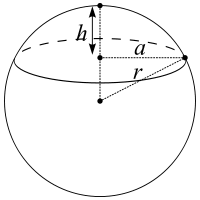The image above is a spherical cap.

To compute the volume of a spherical cap requires two essential parameters which are the radius of the base of the cap (a) and the height (h).

The formula for calculating the volume of the spherical cap:

V = πh(3a² + h²) ⁄ 6

Where;
V = Volume of the spherical cap
a = Radius of the base of the cap
h = Height of the spherical cap

Lets solve an example;
Find the volume of a spherical cap when the radius of the base is 12 cm and the height is 22 cm.

This implies that;
a = Radius of the base of the cap = 12 cm
h = Height of the spherical cap = 22 cm

V = πh(3a² + h²) ⁄ 6
V = 3.142 (22)(3(12)² + 22²) ⁄ 6
V = 3.142 (22)(3(144) + 484) ⁄ 6
V = 3.142 (22)(432 + 484) ⁄ 6
V = 3.142 (22)(916) ⁄ 6
V = 3.142 (20152)6
V = 63309.37 ⁄ 6
V = 10551.56

Therefore, the volume of the spherical cap is 10551.56 cm2.

Nickzom Calculator – The Calculator Encyclopedia is capable of calculating the volume of the spherical cap.

To get the answer and workings of the volume of the spherical cap using the Nickzom Calculator – The Calculator Encyclopedia. First, you need to obtain the app.

You can get this app via any of these means:

To get access to the professional version via web, you need to register and subscribe for NGN 1,500 per annum to have utter access to all functionalities.
You can also try the demo version via https://www.nickzom.org/calculator

Once, you have obtained the calculator encyclopedia app, proceed to the Calculator Map, then click on Mensuration under the Mathematics section.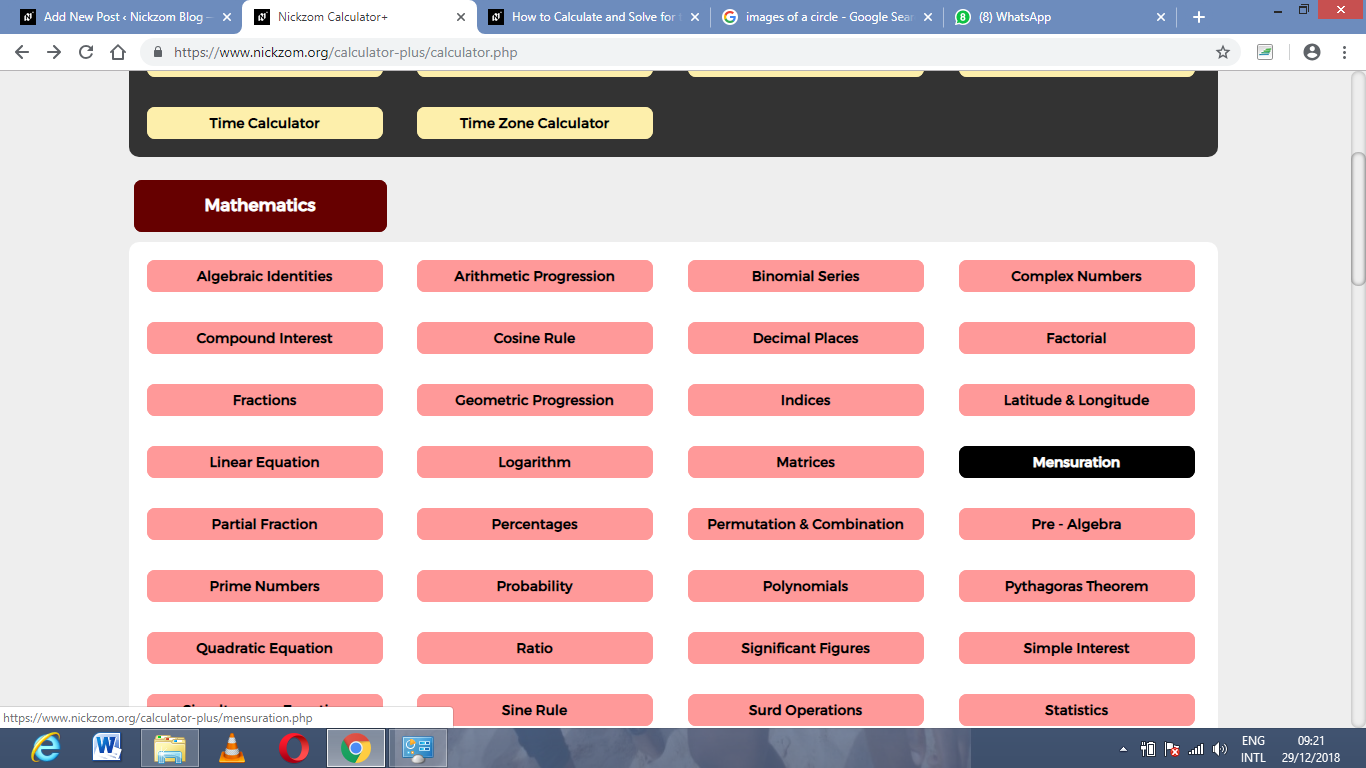Now, click on Volume of the spherical cap under Mensuration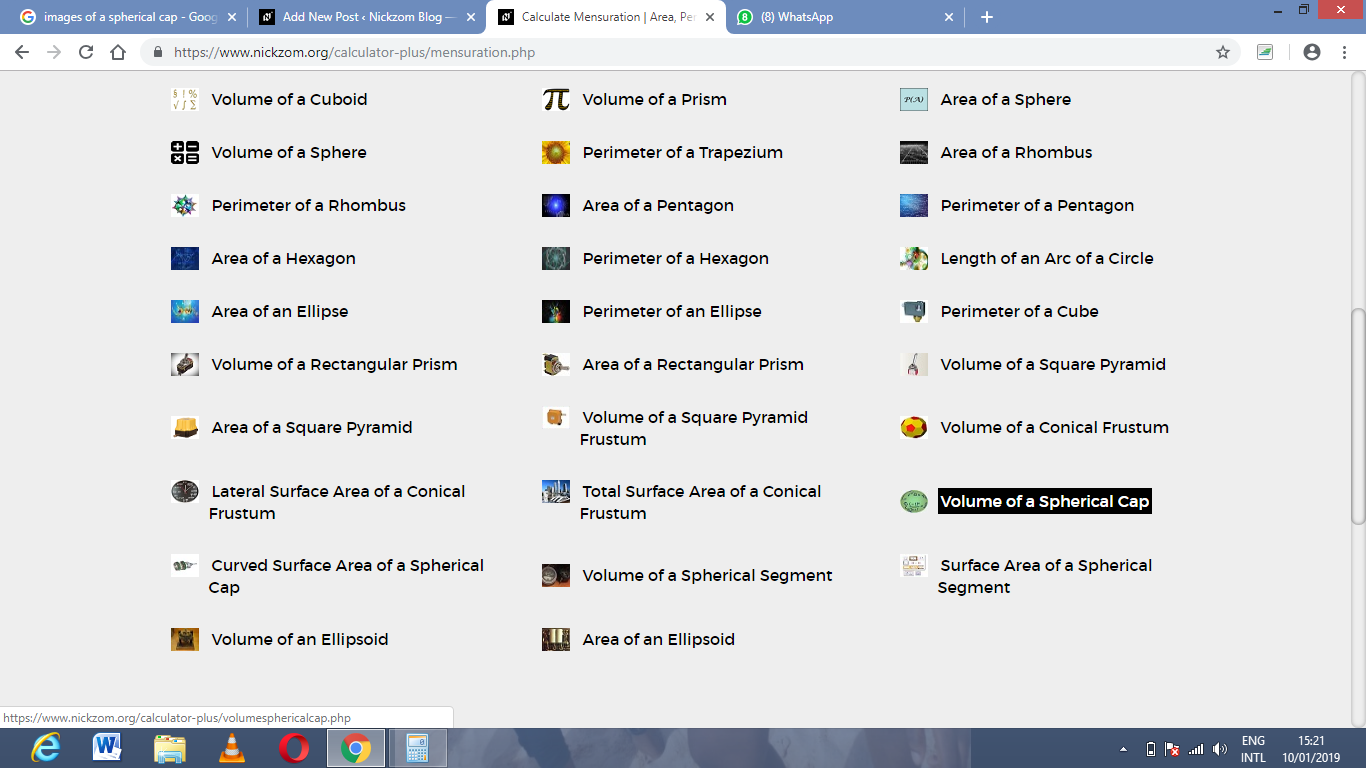The screenshot below displays the page or activity to enter your values, to get the answer for the volume of the spherical cap according to the respective parameters which are the radius of the base of the cap (a) and height (h).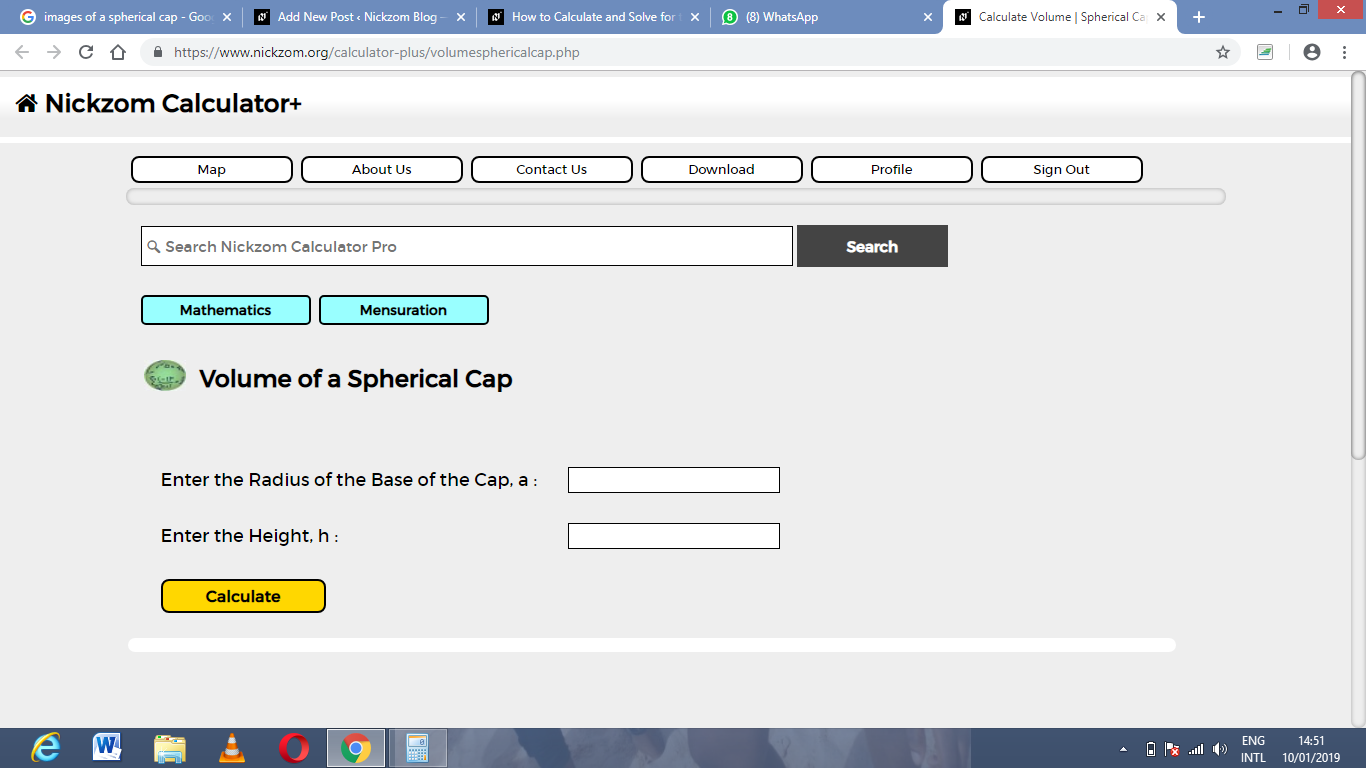Now, enter the values appropriately and accordingly for the parameters as required by the example above where the radius of the base of the cap is 12 cm and the height is 22 cm.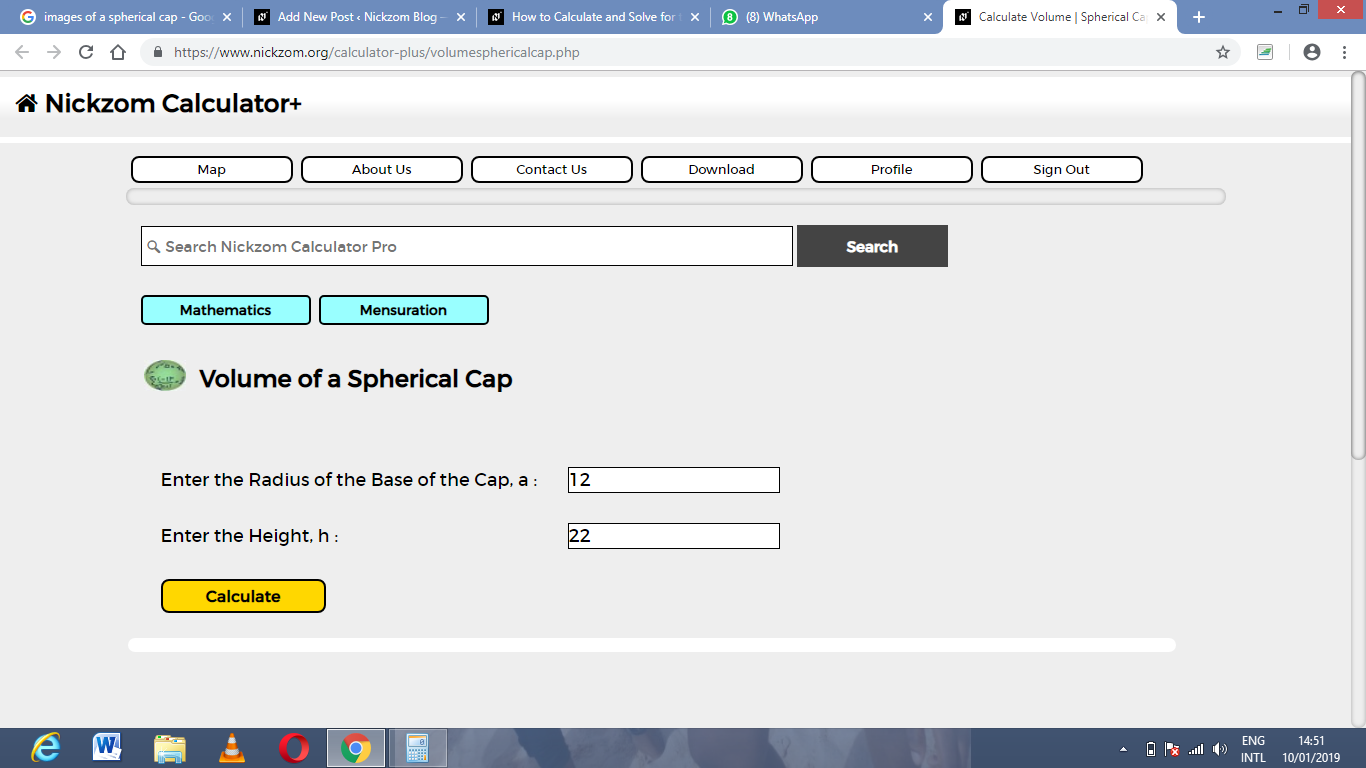Finally, Click on Calculate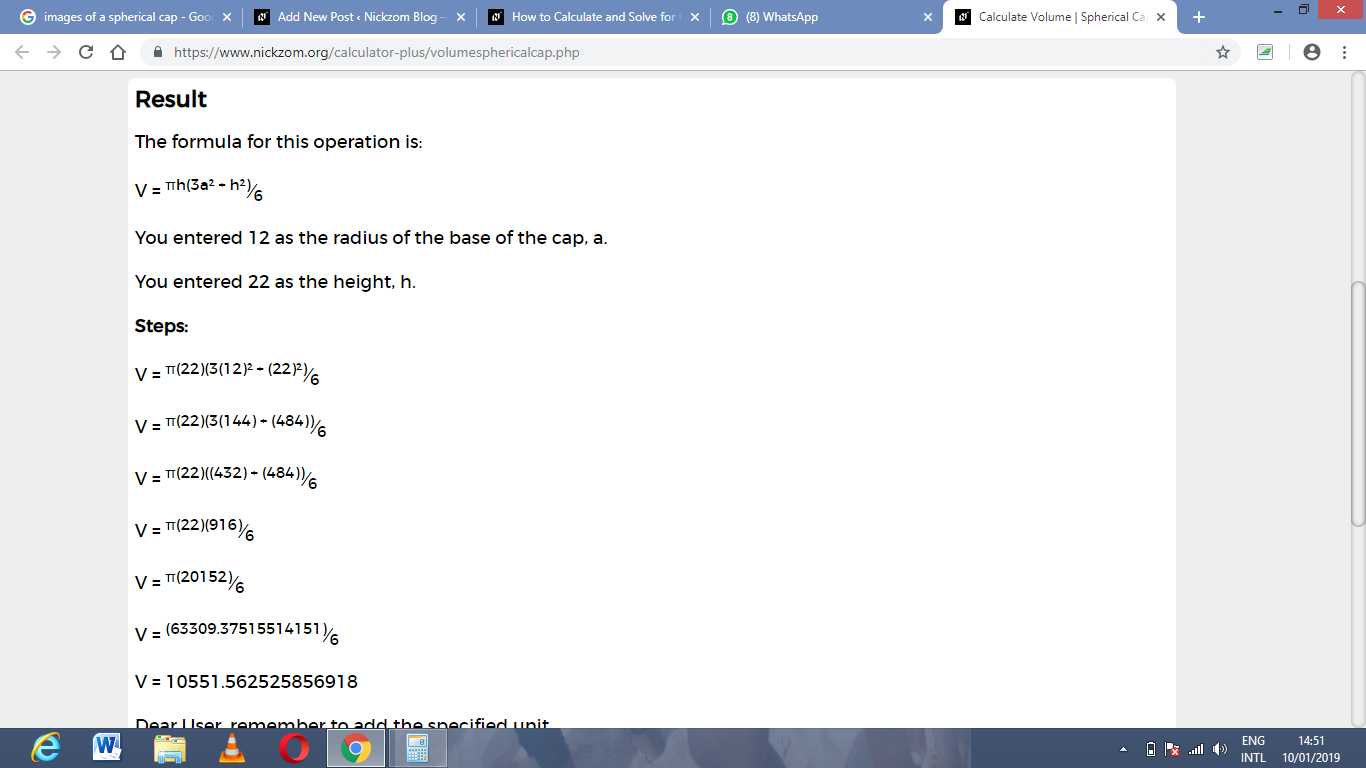As you can see from the screenshot above, Nickzom Calculator – The Calculator Encyclopedia solves for the Volume of a spherical cap and presents the formula, workings and steps too.